Related Articles

# One-Way ANOVA

• Last Updated : 26 Nov, 2020

ANOVA is a parametric statistical technique that helps in finding out if there is a significant difference between the mean of three or more groups. It checks the impact of various factors by comparing groups (samples) on the basis of their respective mean.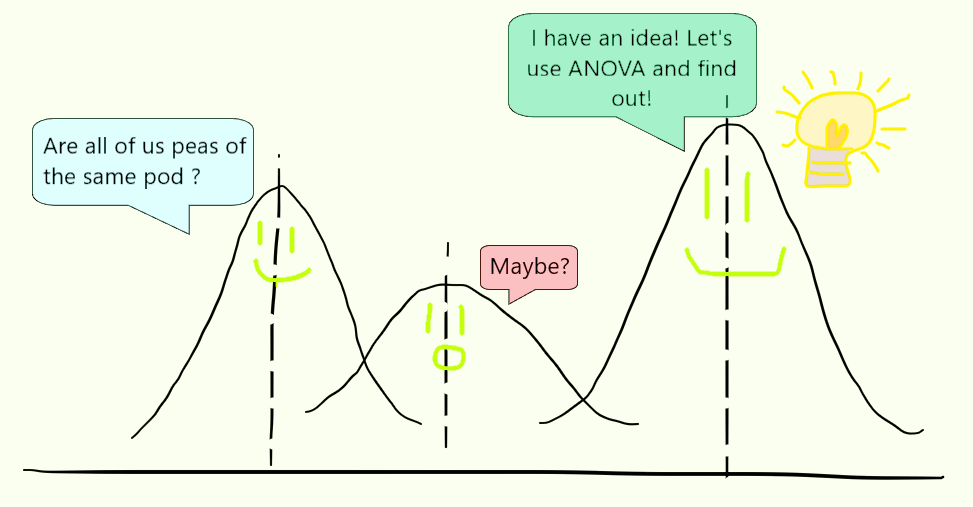We can use this only when:

• the samples have a normal distribution.
• the samples are selected at random and should be independent of one another.
• all groups have equal standard deviations.

One-Way ANOVA

It is a type of hypothesis test where only one factor is considered. We use F-statistic to perform a one-way analysis of variance.

Steps Involved

```Step 1 - Define the null and alternative hypothesis.

H0 -> μ1 = μ2 = μ3 (where μ = mean)
Ha -> At least one difference among the means.
```
```Step 2 - Find the degree of freedom between and within the groups. [Using Eq-1 and Eq-2]
Then find total degree of freedom. [Using Eq-3]
```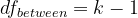Eq-1

```where k = number of groups.
```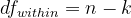Eq-2

```where
n = number of samples in all groups combined.
k = number of groups.
```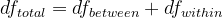Eq-3

For the next step, we need to understand what F-statistic is.

F-value: It is defined as the ratio of the variance between samples to variance within samples. It is obtained while performing ANOVA test. Eq-4 shows the F-value formula for one-way ANOVA.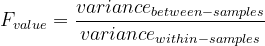Eq – 4

```Step 3 - Refer the F-Distribution table and find Ftable using dfbetween and dfwithin.
As per the given F-Distribution table,
df1 = dfbetween
df2 = dfwithin
[use the given value of α while referring the table.]```
```Step 4 - Find the mean of all samples in each group.
Then use Eq-5 to find the Grand mean. (μGrand)
```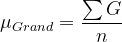Eq – 5

```where,
∑G = sum of all sample values.
n = number of samples.```
```Step 5 - Find the sum of squares total using Eq-6 and sum of squares within using Eq-7.
Then find sum of squares between using Eq-8.
```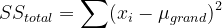Eq-6

```where, xi = ith sample
```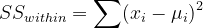Eq-7

```where,
xi = ith sample.
μi = mean of ith group.
```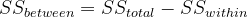Eq-8

```Step 6 - Find the variance (μ2 or S2) between and within samples using Eq-9 and Eq-10.
```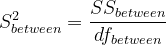Eq-9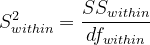Eq-10

```Step 7 - Find Fcalc using Eq-11.
```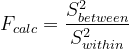Eq-11

Interpreting the results

```if Fcalc < Ftable :
Don't rejct null hypothesis.
μ1 = μ2 = μ3

if Fcalc > Ftable :
Reject null hypothesis.
```

Example Problem

Consider the example given below to understand step by step how to perform this test. The marks of 3 subjects (out of 5) for a group of students is recorded. (as given in the table below)
[Take α = 0.05]

```Step 1 -
Null hypothesis,      H0 -> μE = μM = μS (where μ = mean)
Alternate hypothesis, Ha -> At least one difference among the means of the 3 subjects.```
```Step 2 -
As per the table,
k = 3
n = 9
dfbetween = 3 - 1 = 2 [Eq-1]
dfwithin = 9 - 3 = 6  [Eq-2]
dftotal = 2 + 6 = 8  [Eq-3]```
```Step 3 - On referring to the F-Distribution table (link), using df1 = 2
and df2 = 6 at α = 0.05: we get, Ftable = 5.14
```
```Step 4 - μe = (2 + 4 + 2)/3 = (8/3) = 2.67
μm = (2 + 3 + 4)/3 = (9/3) = 3.00
μs = (1 + 2 + 5)/3 = (8/3) = 2.67

μgrand = (8 + 8 + 9)/9 = (25/9) = 2.78```
```Step 5 - SStotal =  (2 - 2.78)2 + (4 - 2.78)2 + (2 - 2.78)2 +
(2 - 2.78)2 + (3 - 2.78)2 + (4 - 2.78)2 +
(1 - 2.78)2 + (2 - 2.78)2 + (5 - 2.78)2
= 13.60

SSwithin = (2 - 2.67)2 + (4 - 2.67)2 + (2 - 2.67)2 +
(2 - 3.00)2 + (3 - 3.00)2 + (4 - 3.00)2 +
(1 - 2.67)2 + (2 - 2.67)2 + (5 - 2.67)2
= 13.34

SSbetween = 13.60 - 13.34 = 0.23```
```Step 6 - S2between = (0.23/2) = 0.12
S2within = (13.34/6) = 2.22```
```Step 7 - Fcalc = (0.12/2.22) = 0.05
```
```Since, Fcalc < Ftable (0.05 < 5.14)
we cannot reject the null hypothesis. ```

Thus, we can say that the means of all three subjects is the same.

One-way ANOVA compares three or more than three categorical groups to establish whether there is a difference between them. The fundamental strategy of ANOVA is to systematically examine variability within groups being compared and also examine variability among the groups being compared. For any doubt/query, comment below.

Attention reader! Don’t stop learning now. Get hold of all the important Machine Learning Concepts with the Machine Learning Foundation Course at a student-friendly price and become industry ready.

My Personal Notes arrow_drop_up